Finding area by counting squares

Chapter 10 Class 6 Mensuration
Concept wise

We know that

Area is surface inside the figure

But how do we find the area?

We consider a squared paper like this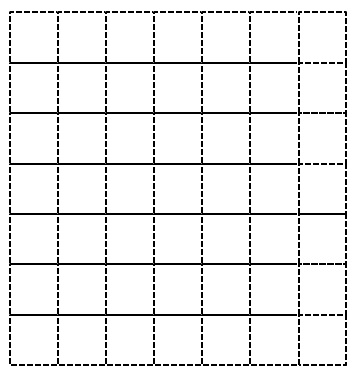In this squared paper,

We make a rectangle like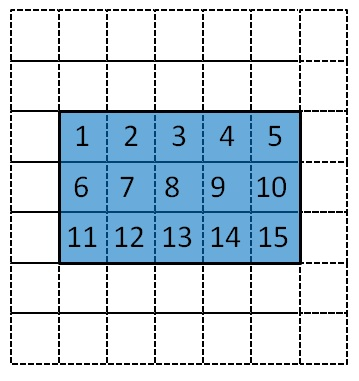To find area, we count the squares inside the rectangle

Number of square = 15

So, Area = 15 square units

Here,

Area will be equal to number of squares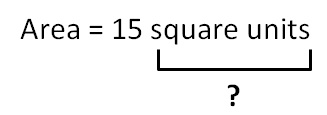#### But what does square units means?

In our figure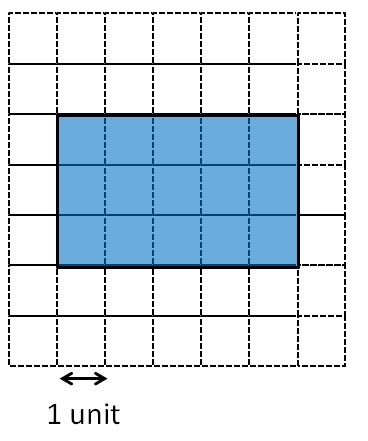Unit means the size of 1 square

Unit can be anything,

cm

m

km

Now,

Square units means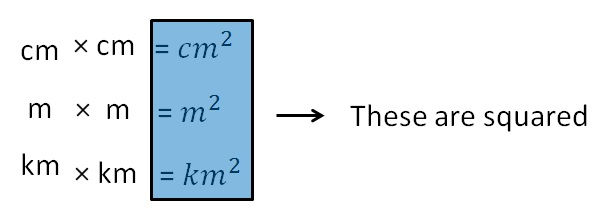While calculating area by counting squares,

• If area is less than half square, ignore it (take it 0)
• If area is more than half square, take it 1 square unit
• If area is exactly half square, take 1/2 square unit

Let’s solve some examples

Let’s find area of this square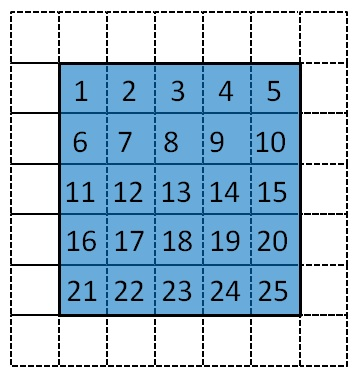Now,  we count the squares

Number of squares = 25

So,

Area = 25 square units

Area of Circle

Let’s find area of circle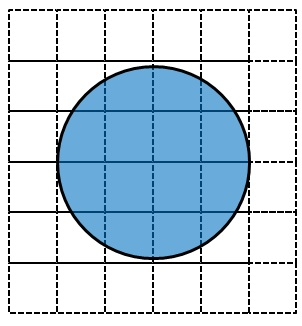Covered Area Number Area Fully - filled squares 4 1 × 4 = 4 Half - filled squares 4 1/2 × 4 = 2 More than Half - filled squares 8 1 × 8 = 8 Less than Half - filled squares 0 0

So,

Area = 4 + 8 + 2

= 14 Square units

Area of Pentagon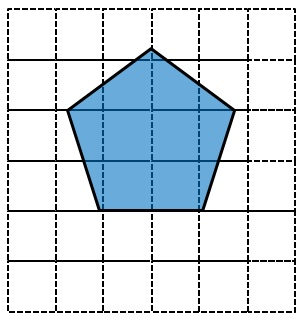Covered Area Number Area Fully - filled squares 4 1 × 4 = 4 Half - filled squares 0 0 More than Half - filled squares 4 1 × 4 = 4 Less than Half - filled squares 6 0

So,

Area = 4 + 4 + 0

= 8 Square units

Learn in your speed, with individual attention - Teachoo Maths 1-on-1 Class